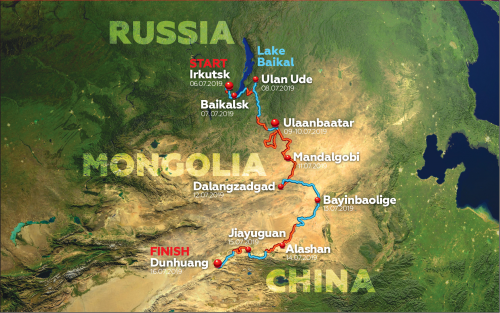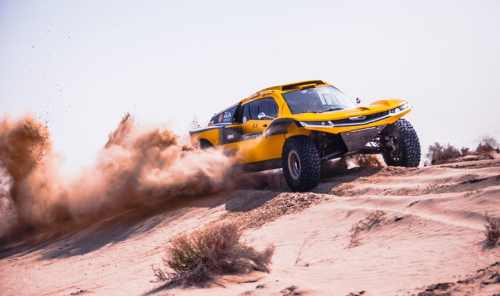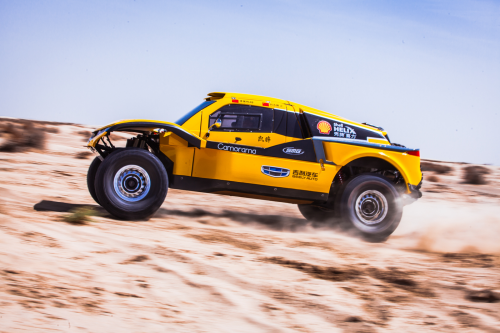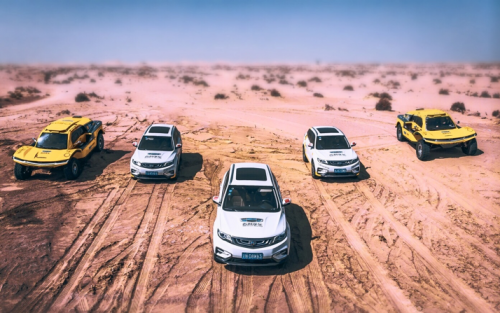## 吉利汽车壳牌润滑油车队即将出征2019丝绸之路拉力赛

2019年07月04日 18:33 来源：车行天下 超过：次关注

【2019年7月2日】 由俄罗斯、蒙古、中国三国携手举办的穿越欧亚大陆的重要洲际越野拉力赛事——2019丝绸之路拉力赛,将于7月6日在俄罗斯伊尔库茨克正式发车。吉利汽车壳牌润滑油车队将再次联手中国最具国际影响力的越野车手韩魏,在国际赛场上展现“中国速度”,助力推广中国汽车赛事运动与赛事文化的发展。2009年,俄罗斯普京总统首次发起并创立了丝绸之路拉力赛。随着2013年9月和10月,中国国家主席习近平分别提出建设“新丝绸之路经济带”和“21世纪海上丝绸之路”的合作倡议,世界各国对共建“一带一路”倡议的认同感和参与度不断增强,丝绸之路拉力赛的影响力也不断扩大。2016年,丝绸之路拉力赛首次走入中国,随着赛事在中俄两国特别是各地方政府的强力支持下,在体育、汽车、能源、经济、人文、旅游等领域与行业产生深远影响,目前已经成为能够比肩达喀尔的重要双周国际赛事。2019丝绸之路拉力赛,由俄罗斯体育部、蒙古国政府、中国国家体育总局主办,国家体育总局汽摩中心、中国汽车摩托车运动联合会、沿途各省(自治区)人民政府承办。同时,本届比赛也是向祖国70周年国庆以及中俄、中蒙两国建交70周年的重要献礼活动,吸引了众多国际顶尖车队和车手参赛。吉利汽车壳牌润滑油车队也将再次出征丝绸之路拉力赛,在国际赛场展现中国速度,进一步印证中国实力。

近年来,吉利集团汽车积极参加国内外重要赛事,助力推动中国赛车文化运动发展。吉利汽车壳牌润滑油车队目前已成为一支国际化、专业化的越野赛车团队。在5月进行的2019环塔拉力赛上,吉利汽车壳牌润滑油车队包揽车队车手双料冠军。作为吉利壳牌润滑油车队的车手,韩魏也不断在国际赛场上展现中国车手的实力,目前已成为中国越野赛车界最顶尖的车手之一。2019年1月,他与领航员廖岷携手征战第41届达喀尔拉力赛,收获了T1.3组别亚军的好成绩。今年的丝绸之路拉力赛将于7月6日从位于贝加尔湖沿线的伊尔库茨克出发,自北向南穿越蒙古并于7月16日抵达中国敦煌。赛程分为10赛段,共计5008公里,其中特殊赛段2593公里;全新线路将为参赛车手带来前所未有的体验和挑战。吉利汽车一直致力于在国际舞台展现中国汽车制造的高水准和高品质。2019丝绸之路拉力赛,吉利汽车被组委会指定为2019丝绸之路拉力赛官方用车,包含博越、缤越、帝豪GS、远景SUV、远景X3在内的共五款、20台车型,将出现在丝绸之路拉力赛赛场中展现“中国实力”。

#### 相关文章

0-500 字已有评论 0条 查看评论>>

﻿
• 快速找车
• 选择品牌
• 选择品牌
• A  奥迪
• A  阿斯顿·马丁
• A  阿尔法·罗密欧
• B  宝沃
• B  布加迪
• B  巴博斯
• B  保时捷
• B  宾利
• B  奔驰
• B  宝马
• B  本田
• B  别克
• B  标致
• B  比亚迪
• B  宝骏
• B  北汽制造
• B  北汽新能源
• B  北汽幻速
• B  北汽威旺
• B  北京汽车
• B  奔腾
• B  北汽绅宝
• C  长安
• C  长安商用
• C  长城
• C  昌河
• D  大众
• D  道奇
• D  DS
• D  东南
• D  东风风神
• D  东风风行
• D  东风小康
• D  东风风度
• D  东风
• F  福特
• F  丰田
• F  菲亚特
• F  法拉利
• F  福田
• F  福迪
• F  福汽启腾
• G  观致
• G  广汽传祺
• G  广汽吉奥
• G  GMC
• H  红旗
• H  汉腾汽车
• H  哈弗
• H  哈飞
• H  海格
• H  海马
• H  华颂
• H  黄海
• H  华泰
• H  恒天
• J  吉利汽车
• J  捷豹
• J  Jeep
• J  江淮
• J  江铃
• J  金杯
• J  九龙
• J  金旅
• K  凯翼
• K  凯迪拉克
• K  克莱斯勒
• K  科尼塞克
• K  卡威
• K  开瑞
• L  路虎
• L  林肯
• L  劳斯莱斯
• L  兰博基尼
• L  雷克萨斯
• L  铃木
• L  雷诺
• L  理念
• L  力帆
• L  莲花汽车
• L  猎豹
• L  路特斯
• L  陆风
• M  马自达
• M  MG
• M  MINI
• M  玛莎拉蒂
• M  摩根
• M  迈凯轮
• N  纳智捷
• O  欧宝
• O  讴歌
• O  欧朗
• Q  奇瑞
• Q  起亚
• Q  启辰
• R  日产
• R  荣威
• R  瑞麒
• S  三菱
• S  斯威汽车
• S  萨博
• S  smart
• S  斯柯达
• S  斯巴鲁
• S  思铭
• S  双龙
• S  上汽大通
• S  双环
• T  特斯拉
• T  腾势
• W  沃尔沃
• W  五菱汽车
• W  五十铃
• W  威兹曼
• W  威麟
• X  现代
• X  雪佛兰
• X  雪铁龙
• X  西雅特
• Y  一汽
• Y  英菲尼迪
• Y  英致
• Y  依维柯
• Y  野马汽车
• Y  永源
• Z  众泰
• Z  中华
• Z  中兴
• Z  知豆
• 选择车系
• 选择车系
• 车型对比
• 选择品牌
• 选择品牌
• A  奥迪
• A  阿斯顿·马丁
• A  阿尔法·罗密欧
• B  宝沃
• B  布加迪
• B  巴博斯
• B  保时捷
• B  宾利
• B  奔驰
• B  宝马
• B  本田
• B  别克
• B  标致
• B  比亚迪
• B  宝骏
• B  北汽制造
• B  北汽新能源
• B  北汽幻速
• B  北汽威旺
• B  北京汽车
• B  奔腾
• B  北汽绅宝
• C  长安
• C  长安商用
• C  长城
• C  昌河
• D  大众
• D  道奇
• D  DS
• D  东南
• D  东风风神
• D  东风风行
• D  东风小康
• D  东风风度
• D  东风
• F  福特
• F  丰田
• F  菲亚特
• F  法拉利
• F  福田
• F  福迪
• F  福汽启腾
• G  观致
• G  广汽传祺
• G  广汽吉奥
• G  GMC
• H  红旗
• H  汉腾汽车
• H  哈弗
• H  哈飞
• H  海格
• H  海马
• H  华颂
• H  黄海
• H  华泰
• H  恒天
• J  吉利汽车
• J  捷豹
• J  Jeep
• J  江淮
• J  江铃
• J  金杯
• J  九龙
• J  金旅
• K  凯翼
• K  凯迪拉克
• K  克莱斯勒
• K  科尼塞克
• K  卡威
• K  开瑞
• L  路虎
• L  林肯
• L  劳斯莱斯
• L  兰博基尼
• L  雷克萨斯
• L  铃木
• L  雷诺
• L  理念
• L  力帆
• L  莲花汽车
• L  猎豹
• L  路特斯
• L  陆风
• M  马自达
• M  MG
• M  MINI
• M  玛莎拉蒂
• M  摩根
• M  迈凯轮
• N  纳智捷
• O  欧宝
• O  讴歌
• O  欧朗
• Q  奇瑞
• Q  起亚
• Q  启辰
• R  日产
• R  荣威
• R  瑞麒
• S  三菱
• S  斯威汽车
• S  萨博
• S  smart
• S  斯柯达
• S  斯巴鲁
• S  思铭
• S  双龙
• S  上汽大通
• S  双环
• T  特斯拉
• T  腾势
• W  沃尔沃
• W  五菱汽车
• W  五十铃
• W  威兹曼
• W  威麟
• X  现代
• X  雪佛兰
• X  雪铁龙
• X  西雅特
• Y  一汽
• Y  英菲尼迪
• Y  英致
• Y  依维柯
• Y  野马汽车
• Y  永源
• Z  众泰
• Z  中华
• Z  中兴
• Z  知豆
• 选择车系
• 选择车系
• 选择车型
• 选择车型
• 意见反馈